•曲线拟合
• 用EMGU_OpenCV的矩阵实现的多项式拟合计算，得到曲线参数。可以更改代码实现不同高次多项式曲线拟合
• 2. 用高阶多项式函数拟合曲线； 3. 用解析解求解两种loss的最优解（无正则项和有正则项） 4. 优化方法求解最优解（梯度下降，共轭梯度）； 5. 用你得到的实验数据，解释过拟合。 6. 用不同数据量，不同超参数，不同...
• 对于多维的两组同维数据，可以反复调用此函数，处理大批量类似问题
• Matlab 多项式曲线拟合polyfit

万次阅读 多人点赞 2018-08-18 12:01:04
多项式曲线拟合 常见语法  a = polyfit ( x, y, n) 说明  a=polyfit(x,y,n)中参数n为x的最高阶,返回值a是n阶函数的系数,a是一个长度为n+1的行向量， 即拟合出来的公式形式应为：  示例 用多项式...

polyfit

多项式曲线拟合

常见语法

a = polyfit ( x, y, n)

说明

a=polyfit(x,y,n)中参数n为x的最高阶,返回值a是n阶函数的系数,a是一个长度为n+1的行向量，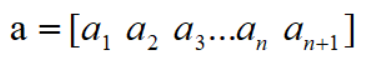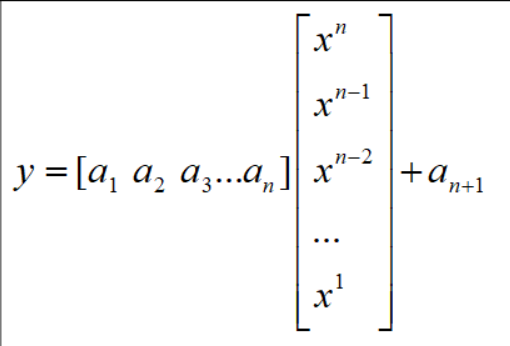即拟合出来的公式形式应为：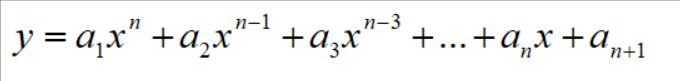示例

用多项式拟合法求一个形如 的公式，使它与表1.1中所列数据拟合

 x 19 25 31 38 44 y 19 32.3 49 73.3 97.8

解: 编写程序如下

>> x=[19 25 31 38 44];
y=[19.0 32.3 49.0 73.3 97.8];
a=polyfit(x,y,2);          %拟合2次函数
x0=19:0.1:44;              %步长为0.1
y0=polyval(a,x0);          %返回值y0是对应于x0的函数值
plot(x,y,'o',x0,y0,'r')    %画图，o表示圆圈，r表示红色red
legend('拟合前','拟合后')   %给曲线加上说明
xlabel('x');               %给x轴加上说明
ylabel('y');
grid on;                   %添加网格线
set(gca,'GridLineStyle',':','GridColor','k','GridAlpha',1);  %将网格线变成虚线
a                          %直接输出a

a =

0.0497    0.0193    0.6882

所以可以得出拟合函数

效果如下图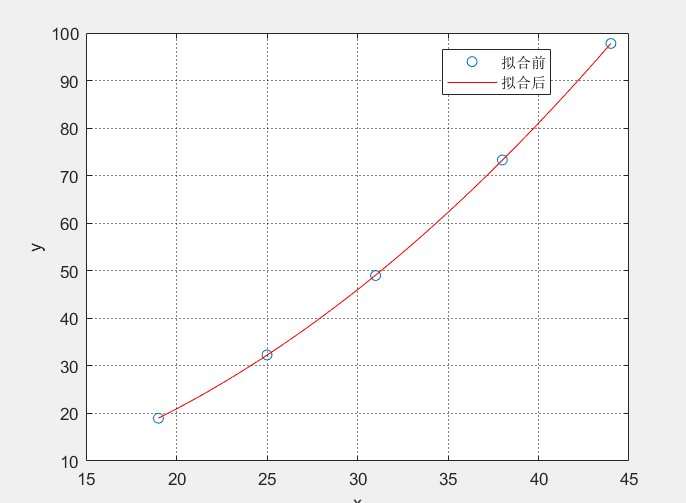展开全文matlab polyfit 多项式曲线拟合
• 用勒让德多项式技术拟合复刻输入信号，使其拟合的输入信号准确性提高从而使整个系统精度有所提升
• 该程序找到 2 个多项式，Num(x) 和 Den(x)，使得 Num(xi)/Den(xi) = y(xi) 在最小二乘意义上。 函数 y(xi) 可能具有无限值和零值。 这是对 Matlab 的 Polyfit 命令的升级。matlab
• 主要介绍了Apache Commons Math3探索之多项式曲线拟合实现代码，小编觉得挺不错的，这里分享给大家，供需要的朋友参考。
• 函数基础逼近算法，对离散试验数据点的多项式曲线拟合
• 曲线拟合的三种功能: (1)估算数据 (2)预测趋势 (3)总结规律 1、引例-人口预测问题 人口增长是当今世界上都关注的问题，对人口增长趋势进行预测是各国普遍的做法。已知某国1790年到2010年间历次人口普查数据如下表所...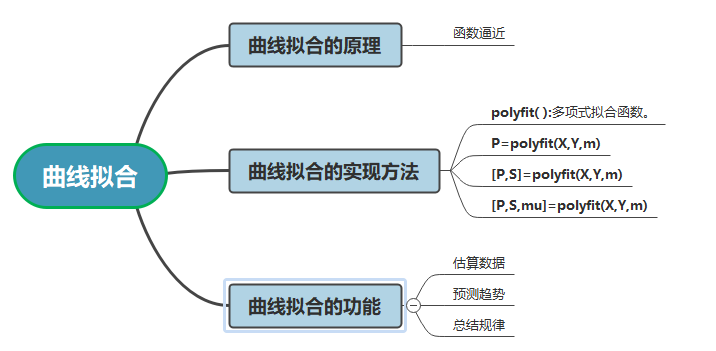曲线拟合的三种功能:
(1)估算数据
(2)预测趋势
(3)总结规律

1、引例-人口预测问题
人口增长是当今世界上都关注的问题，对人口增长趋势进行预测是各国普遍的做法。已知某国1790年到2010年间历次人口普查数据如下表所示，请预测该国2020年的人口数。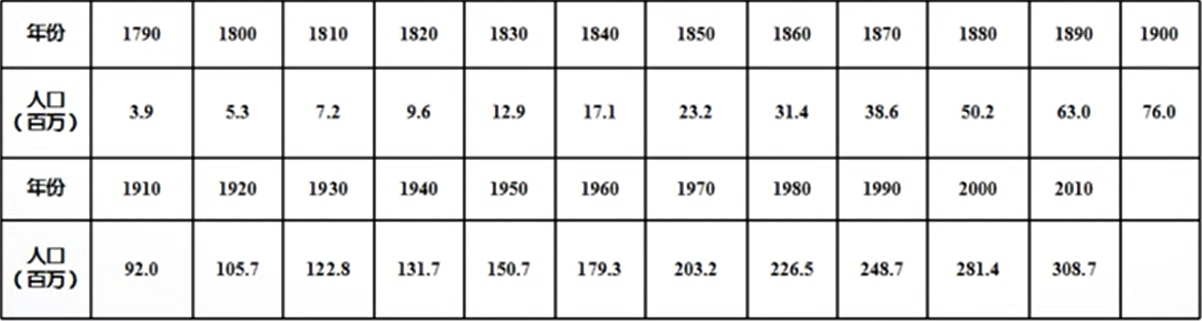解决思路:
找一个函数，去逼近这些数据，然后再根据找到的函数，计算预测点的值。

polyfit()： 用于建立多项式函数去逼近样本数据

x = 1790:10:2010;     %时间向量
y = [3.9 5.3 7.2 9.6 12.9 17.1 23.2 31.4 38.6 50.2 63 76 92 ...
105.7 122.8 131.7 150.7 179.3 203.2 226.5 248.7 281.4 308.7];   %人口向量

p = polyfit(x,y,3);    %生成3次多项式函数，将系数向量保存在p中
polyval(p,2020)        %计算该多项式函数在2020处的函数值,结果为339.8
plot(x,y,'*',x,polyval(p,x),'r')
legend('散点图','拟合曲线','location','southeast')
title('人口预测问题','color','r','fontsize',14)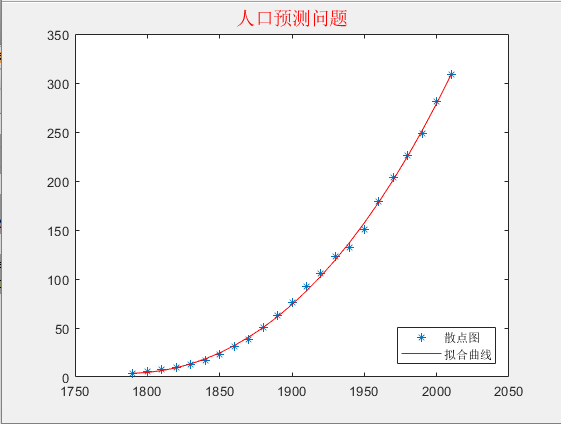2、曲线拟合的原理
与数据插值类似，曲线拟合也是一种函数逼近的方法。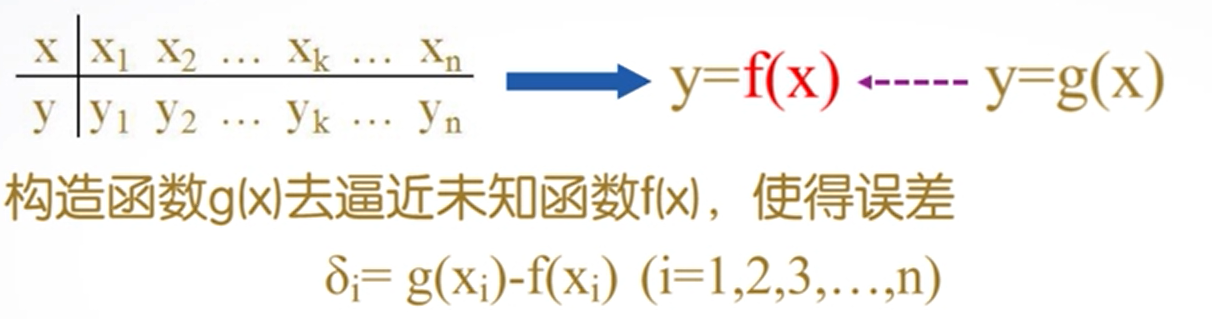在某种意义下达到最小。两个问题:
(1)用什么类型的函数做逼近函数?

多项式函数能够将复杂阃题简单化，表达能力强、计算方便、函数性态好。
(2）误差最小到底怎么计算?
最小二乘法（又称最小平方法)是一种数学优化技术。它通过最小化误差的平方和来寻找数据的最佳函数匹配。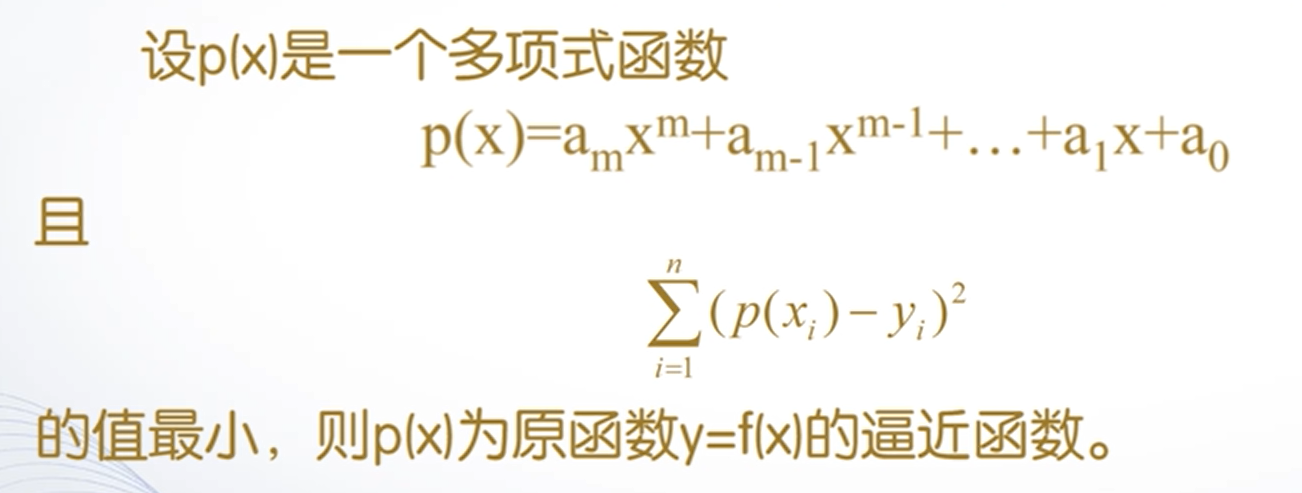3、曲线拟合的实现方法
polyfit( ):多项式拟合函数。
函数功能:求得最小二乘拟合多项式系数。调用格式:
( 1) P=polyfit(X,Y,m)
( 2)[P,S]=polyfit(X,Y,m)
(3 )[P,S,mu]=polyfit(X,Y,m)

根据样本数据X和Y，产生一个m次多项式P及其在采样点误差数据S，mu是一个二元向量，mu(1)是mean(X)（平均值）,而mu(2)是std(X)（标准差）。

x = 1950:10:2010;     %时间向量,二战后至今
y = [150.7 179.3 203.2 226.5 248.7 281.4 308.7];   %人口向量

p = polyfit(x,y,3);   %生成3次多项式函数，将系数向量保存在p中，发现前两个系数为0，拟合次数太高
p = polyfit(x,y,2)
plot(x,y,'*',x,polyval(p,x),'r')
legend('散点图','拟合曲线','location','southeast')
title('人口预测问题','color','r','fontsize',14)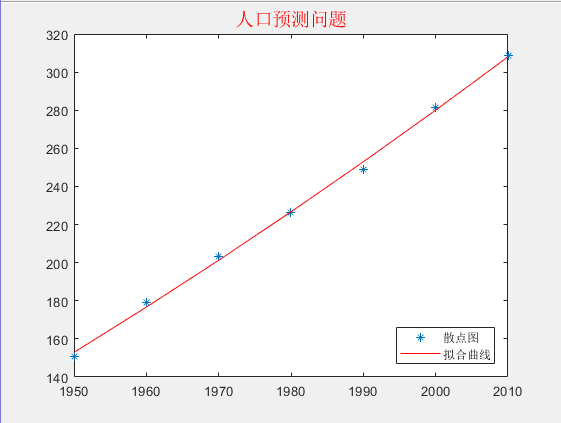在利用曲线拟合解决实际问题时
（1）要对问题的背景进行详细的分析。
（2）采样点并非越多越好，适当的时候可以减少采样点,分段进行拟合。

展开全文matlab 曲线拟合
• 1、什么是多项式曲线拟合 多项式拟合就是我们需要得到一个无限逼近真实曲线的的多项式： y(x,W)=w0+w1x+w2x2+…+wkxk=∑i=0kwixi y(x,W) = w_0 + w_1x + w_2x^2 + … + w_kx^k = \sum_{i=0}^{k}w_ix^i y(x,W)=w0​+...

1、什么是多项式曲线拟合

多项式拟合就是我们需要得到一个无限逼近真实曲线的的多项式：
y ( x , W ) = w 0 + w 1 x + w 2 x 2 + … + w k x k = ∑ i = 0 k w i x i y(x,W) = w_0 + w_1x + w_2x^2 + … + w_kx^k = \sum_{i=0}^{k}w_ix^i
注：

k k 为多项式的阶数

w 0 , … , w k w_0,…,w_k 为多项式的系数，记为 W W

使用均方误差作为误差函数对拟合出的多项式进行评估，公式如下：
E ( W ) = 1 2 ∑ i = 1 n ( y ( x i , W ) − t n ) 2 = 1 2 ( X W − T ) T ( X W − T ) E(W) = \frac{1}{2} \sum_{i=1}^{n}(y(x_i,W)-t_n)^2=\frac{1}{2} (XW-T)^T(XW-T)
其中：
W = [ w 0 w 1 ⋮ w k ] ， X = [ 1 x 1 ⋯ x 1 k 1 x 2 ⋯ x 2 k ⋮ ⋮ ⋱ ⋮ 1 x n ⋯ x n k ] W =\begin{bmatrix} w_0 \\ w_1 \\ \vdots \\ w_k \\ \end{bmatrix}，X=\begin{bmatrix} 1 & x_1 & \cdots & x_1^k \\ 1 & x_2 & \cdots & x_2^k \\ \vdots & \vdots & \ddots & \vdots \\ 1 & x_n & \cdots & x_n^k \\ \end{bmatrix}
注：

n n 为样本的点数

k k 为拟合出的多项式的阶数

拟合数据的目的即为最小化误差函数，因为误差函数是多项式系数W的二次函数，所以存在唯一最小值，且在导数为零处取得。对W求导并令导数为零得到：
∂ E ( W ) ∂ W = X T X W − X T T W = ( X T X ) − 1 X T T \frac{\partial E(W)}{\partial W} = X^TXW-X^TT \\ W = (X^TX)^{-1}X^TT
故可以通过矩阵运算得到W。

2、泛化能力

泛化能力（generalization ability）是指机器学习算法对新鲜样本的适应能力，简而言之是在原有的数据集上添加新的数据集，通过训练输出一个合理的结果。学习的目的是学到隐含在数据背后的规律，对具有同一规律的学习集以外的数据，经过训练的网络也能给出合适的输出，该能力称为泛化能力。

2.1、欠拟合

欠拟合（under-fitting）是指模型拟合程度不高，数据距离拟合曲线较远，模型没有很好地捕捉到数据特征，不能够很好地拟合数据。

2.2、过拟合

过拟合（over-fitting）其实就是所建的机器学习模型或者是深度学习模型在训练样本中表现得过于优越学到了很多没必要的特征，导致在验证数据集以及测试数据集中表现不佳。

3、如何对拟合结果做出评估

3.1、使用拟合优度指数进行评价

y y 为待拟合数值，其均值为 y ˉ \bar y ，拟合值为 y ^ \hat y ，则：

总平方和（ S S T SST ）:
S S T = ∑ i = 1 n ( y i − y ˉ ) 2 SST = \sum_{i=1}^{n}(y_i- \bar y)^2
回归平方和（ S S E SSE ）：
S S R = ∑ i = 1 n ( y ^ i − y ˉ ) 2 SSR = \sum_{i=1}^{n}(\hat y_i- \bar y)^2
残差平方和（ S S E SSE ）实际值与预测值之间差的平方之和：
S S E = ∑ i = 1 n ( y i − y ^ i ) 2 SSE = \sum_{i=1}^{n}(y_i - \hat y_i)^2
则有：
S S T = S S R + S S E SST = SSR + SSE
决定系数（ R 2 R^2 ）:
R 2 = S S R S S T = ∑ i = 1 n ( y ^ i − y ˉ ) 2 ∑ i = 1 n ( y i − y ^ i ) 2 = 1 − S S E S S T R^2 = \frac{SSR}{SST} = \frac{\sum_{i=1}^{n}(\hat y_i- \bar y)^2}{\sum_{i=1}^{n}(y_i - \hat y_i)^2} = 1 - \frac{SSE}{SST}
通常认为，用 y ˉ \bar y 去预测 y y 是一个最差情况（实际上可能比这个还差，比如实际值都是 1 1 ，预测值却都是 10000 10000 ），任何预测都应该比这个准。

于是 R 2 = 1 − S S E S S T R^2 = 1 - \frac{SSE}{SST} （也就是预测值和真实值的残差平方和）要小于平均值和真实值的残差平方和，即 S S E < S S T SSE<SST R 2 R_2 一般介于 0 0 1 1 之间，越大拟合效果越好，但如果模型出乎意料的非常差，可能为负。

4、利用正则化改善过拟合

最小化误差函数用于衡量对于任意给定的 w w 值，函数 y ( x , W ) y(x,W) 与训练数据的差异（即将 x x 带入函数 y ( x , W ) y(x,W) 得到的值与训练数据集中相应的值得差异）。

其中，误差函数为：
E ( W ) = 1 2 ∑ n = 1 N { y ( x n , W ) − t n } 2 E(W) = \frac{1}{2} \sum_{n=1}^{N} \{y(x_n,W)-t_n \}^2
注：

1 2 \frac{1}{2} 是为了方便计算引入的

N N 为样本的个数

可以通过选择使得 E ( w ) E(w) 尽可能小的 w w 来解决曲线拟合问题。

由于误差函数是系数 w w 的二次函数，因此它关于系数的导数是 w w 的线性函数，所以误差函数的最小值有一个唯一解，记作 W ∗ W∗ ，可以用解析的方式求出。最终的多项式函数由函数 y ( x , W ∗ ) y(x, W∗) 给出。

在定义误差函数是增加惩罚项（这种惩罚项最简单的形式采用所有系数的平方和），使多项式系数被有效控制，不会过大。

则惩罚项为：
∣ ∣ w ∣ ∣ 2 = w T w = w 0 2 + w 1 2 + … + w k 2 ||w||^2 = wTw = {w_0}^2 + {w_1}^2 + … + {w_k}^2
新定义的误差函数为：
E ~ ( w ) = 1 2 ∑ n = 1 N { y ( x n , w ) − t n } 2 + λ 2 ∣ ∣ w ∣ ∣ 2 \tilde{E}(w) = \frac{1}{2} \sum_{n=1}^{N} \{y(x_n,w)-t_n \}^2+\frac{\lambda}{2}||w||^2
注：

系数 λ \lambda 控制了正则化项相对于平方和误差项的重要性，被称之为正则化系数。

求导置零得到：
W = ( X T X + λ E m + 1 ) − 1 X T T W=(X^TX+\lambda E_{m+1})^{-1}X^TT

W W 为多项式的系数

通过改变 λ \lambda 的大小（如： λ \lambda 较小时 l n λ = − 18 ln\lambda = − 18 λ \lambda 较大时 l n λ = − 0 ln\lambda = − 0 ））可以降低多项式的阶数，防止过拟合。

代码实现

import matplotlib.pyplot as plt
import numpy as np
from sklearn.linear_model import Ridge
from sklearn.metrics import r2_score
from sklearn.preprocessing import PolynomialFeatures

from PolynomialFitting.PolynomialFittingTest import PolynomialFittingTest

class PolynomialFitting:
@staticmethod
def fitting(x_list, y_list, degree=2):
"""
拟合
:param y_list:Y坐标
:param x_list: X坐标
:param degree: 阶数
:return:w参数矩阵, r2拟合优度指数, f可以参与计算的多项式
"""
# 参数格式效验
if not isinstance(x_list, list):
raise Exception(print("X坐标参数类型错误"))
if not isinstance(y_list, list):
raise Exception(print("Y坐标参数类型错误"))
if not isinstance(degree, int):
raise Exception(print("多项式阶数错误"))

# 将list装换为ndarray
try:
x_array = np.array(x_list)
y_array = np.array(y_list)
except:
raise Exception(print("坐标转换失败"))

# 对X坐标和Y坐标的形状进行比较，不一致则无法完成拟合
x_shape = x_array.shape
y_shape = y_array.shape
if x_shape != y_shape:
raise Exception("X坐标与Y坐标无法对应")

# w为拟合后的多项式的系数
try:
w = np.polyfit(x_array, y_array, degree)
except:
raise Exception("多项式阶数过高")

f = PolynomialFitting.get_fx(w)
r2 = PolynomialFitting.get_r2(y_array, x_array, f)

return w, r2, f

@staticmethod
def fitting_with_lambda(x_list, y_list, degree=2, lambda_=0.001):
"""
正则化拟合（利用岭回归为其添加惩罚项）
:param x_list: 训练集的X坐标
:param y_list: 训练集的Y坐标
:param degree: 要拟合的多项式的阶数
:param lambda_:
:return:
"""
# 参数格式效验
if not isinstance(x_list, list):
raise Exception(print("X坐标参数类型错误"))
if not isinstance(y_list, list):
raise Exception(print("Y坐标参数类型错误"))
if not isinstance(degree, int):
raise Exception(print("多项式阶数错误"))
if not isinstance(lambda_, float):
raise Exception(print("lambda系数错误"))

if lambda_ <= 0.0:
raise Exception(print("非法的lambda值"))

# 将list装换为ndarray
try:
x_array = np.array([x_list])
y_array = np.array([y_list])
except:
raise Exception(print("坐标转换失败"))

# 对X坐标和Y坐标的形状进行比较，不一致则无法完成拟合
x_shape = x_array.shape
y_shape = y_array.shape
if x_shape != y_shape:
raise Exception("X坐标与Y坐标无法对应")

# 矩阵转置
x_array = x_array.T
y_array = y_array.T

# 设置多项式的阶数
poly = PolynomialFeatures(degree=degree)

x_list_ploy = poly.fit_transform(x_array)

# Ridge(岭回归)通过对系数大小施加惩罚来解决拟合的一些问题。
# alpha >= 0 是控制系数收缩量的复杂性参数，alpha值越大，收缩量越大，模型对共线性的鲁棒性也更强。
lr = Ridge(alpha=(lambda_ / 2))
# Ridge 用 fit 方法完成拟合，并将模型系数 w 存储在其 coef_ 成员中
lr.fit(x_list_ploy, y_array)
# 获取多项式系数(参数从低向高)
w = lr.coef_
# 逆序
w_l = w.tolist()
w_l.reverse()
w = np.array(w_l)

# 获取可以参与计算的多项式表达式
f = PolynomialFitting.get_fx(w)
# 计算r2拟合优度指数
r2 = PolynomialFitting.get_r2(y_list, x_list, f)
return w, r2, f

@staticmethod
def print_polynomial(w_list):
"""
获取多项式
:param w_list: 参数列表
:return:
"""
fx = "y = "
for i in range(0, len(w_list)):
param = w_list[i]
order = len(w_list) - 1 - i
if order:
fx += "{} * x ^ {} + ".format(param, order)
else:
fx += "{}".format(param)
return fx

@staticmethod
def get_fx(w_list):
"""
获取可以参与计算的多项式
:param w_list:w参数矩阵
:return:可以参与计算的多项式
"""
f = np.poly1d(w_list)
return f

@staticmethod
def get_r2(y_ture, x_ture, f):
"""
计算拟合优度指数
:param y_ture:Y坐标真实值
:param x_ture:X坐标真实值
:param f:可以参与计算的多项式
:return:r2拟合优度指数
"""
# 计算R2
# coefficient_of_determination = r2_score(y_ture, f(x_ture))
r2 = r2_score(y_ture, f(x_ture))
return r2

@staticmethod
def get_best_fitting(x_list, y_list):
"""
获取最优拟合结果
:param x_list: X坐标数组
:param y_list: Y坐标数组
:return:
"""
degree = 1
best_degree = 1
w_r, r2_r, f_r = PolynomialFitting.fitting(x_list, y_list, degree)
while True:
try:
# 多项式拟合
w, r2, f = PolynomialFitting.fitting(x_list, y_list, degree)
# print("多项式参数列表：{}".format(w))
# print("多项式阶数：{}".format(order))
# print("拟合优度指数：{}".format(r2))
print("阶数：{}\t拟合优度指数：{}".format(degree, r2))

if r2 <= 0 or r2 > 1:
break

if w == 0:
degree += 1
continue

if r2 > r2_r:
w_r = w
r2_r = r2
f_r = f
best_degree = degree

degree += 1
except:
break

# time.sleep(0.5)
print("正常结束")
return w_r, r2_r, f_r, best_degree

@staticmethod
def get_best_fitting_with(x_real, y_real):
best_degree = 2
w_r, r2_r, f_r = PolynomialFitting.fitting_with_lambda(x_real, y_real)
lambda_r = np.exp(0)

for degree in range(2, 200):
for i in range(0, -19, -1):
lambda_ = np.exp(i)
w, r2, f = PolynomialFitting.fitting_with_lambda(x_real, y_real, degree=degree, lambda_=lambda_)
print("多项式阶数：{},lambda系数：{}".format(degree, lambda_))

# print("多项式参数列表：{}".format(w_2))
# print("多项式阶数：{}".format(degree))
# print("lambda系数：{}".format(lambda_))
# print("拟合优度指数：{}".format(r2_2))
if r2 > r2_r:
w_r = w
r2_r = r2
f_r = f
best_degree = degree
lambda_r = lambda_

return w_r, r2_r, f_r, best_degree, lambda_r

if __name__ == '__main__':
# 生成测试数据
X_train, y_train = PolynomialFittingTest.create_data(100)
print(X_train)
print(y_train)
x_l = X_train.reshape((1, 100)).tolist()
y_l = y_train.reshape((1, 100)).tolist()

# 未考虑正则化
w_1, r2_1, f1, best_degree_1 = PolynomialFitting.get_best_fitting(x_l, y_l)
print("多项式参数列表：{}".format(w_1))
print("多项式阶数：{}".format(best_degree_1))
print("拟合优度指数：{}".format(r2_1))

# 考虑正则化
w_2, r2_2, f2, best_degree_2, lambda_2 = PolynomialFitting.get_best_fitting_with(x_l, y_l)
print("多项式参数列表：{}".format(w_2))
print("多项式阶数：{}".format(best_degree_2))
print("lambda系数：{}".format(lambda_2))
print("拟合优度指数：{}".format(r2_2))

# 绘制画布
plt.figure()
# 解决中文显示问题
plt.rcParams['font.sans-serif'] = ['SimHei']
plt.rcParams['axes.unicode_minus'] = False
# 绘制散点图
plt.scatter(x_l, y_l)

# 常规拟合
y_pre_1 = f1(x_l)
plt.plot(x_l, y_pre_1, color='b', label="常规拟合")

# 绘制岭回归曲线
y_pre_2 = f2(x_l)
plt.plot(x_l, y_pre_2, color='r', label="岭回归")

# 真实曲线
y_sin = PolynomialFittingTest.sin_fun(X_train)
plt.plot(x_l, y_sin, color='g', label="$sin(x)$")

# 设置图片标题
plt.title("预测曲线")
# 显示图例
plt.legend()
plt.show()

参考资料

[Python] 多项式曲线拟合(Polynomial Curve Fitting)：https://blog.csdn.net/m0_38068229/article/details/105202554

机器学习入门之多项式曲线拟合：https://blog.csdn.net/xwl198937/article/details/52210156

Numpy实现多项式曲线拟合：https://www.cnblogs.com/zhjblogs/p/14725864.html

numpy进行多项式拟合：https://www.cnblogs.com/yjybupt/p/12972682.html

过拟合和欠拟合：https://blog.csdn.net/weixin_42575020/article/details/82949285

泛化能力_百度百科：https://baike.baidu.com/item/%E6%B3%9B%E5%8C%96%E8%83%BD%E5%8A%9B/3323240

欠拟合_百度百科：https://baike.baidu.com/item/%E6%AC%A0%E6%8B%9F%E5%90%88/22692155

多项式曲线拟合：https://zhuanlan.zhihu.com/p/53056358

展开全文机器学习 python 人工智能 过拟合 多项式拟合
• 多项式拟合在机器学习中有着重要的应用，下面我们通过一个案例来说明如何进行多项式曲线拟合以及拟合中会遇到的问题。内容主要包含了：问题定义拟合正则化总结需要使用到的Python库有：import matplotlib.pyplot as ...多项式拟合在机器学习中有着重要的应用，下面我们通过一个案例来说明如何进行多项式曲线拟合以及拟合中会遇到的问题。内容主要包含了：

• 问题定义
• 拟合
• 正则化
• 总结

需要使用到的Python库有：

import matplotlib.pyplot as plt
import numpy as np
from sklearn.linear_model import LinearRegression
from sklearn.linear_model import Ridge
from sklearn.preprocessing import PolynomialFeatures

%matplotlib inline

问题定义

首先假设我们的训练集由次观测得到，为均匀分布于区间[0,1]。对应的观测集为，实际的目标函数为。其中训练集是已知的，我们的目的是通过训练集和观测集拟合出预测函数，让它尽可能的接近目标函数，所以生成观测集的方式是通过训练集加上随机噪声输入到目标函数得到。因为在现实问题中获取到的训练数据也是夹杂了各种噪声，所以这样的拟合对于实验更有意义。

先来看一个的曲线图：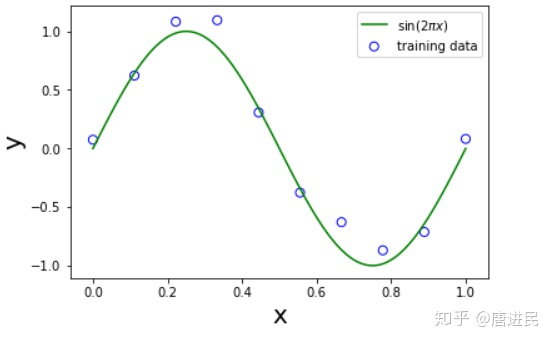代码如下：

def

绿色的曲线就是我们要拟合的目标函数，接下来我们使用多项式函数来拟合我们的数据，多项式定义为：其中是多项式的阶数，是多项式的系数，记做，可以看到虽然多项式函数是关于非线性函数，但是却是关于多项式系数的线性函数。有了多项式以后我们还需要一个误差函数来对我们拟合出的多项式进行评估，这里我们使用均方误差，公式如下：接下来就可以对数据进行拟合了。

拟合

我们拟合数据的目的是最小化误差函数，因为误差函数是多项式系数的二次函数，因此它关于系数的导数是线性函数，所以误差函数的最小值有一个唯一解，我们记做，下面我们通过选择不同的阶来对数据进行拟合，得到结果如下：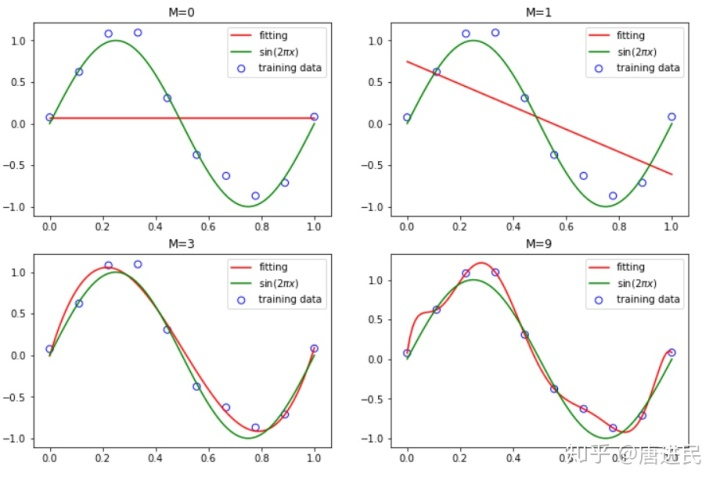代码如下：

fig = plt.figure(figsize=(12,8))
for i,order in enumerate([0,1,3,9]):
plt.subplot(2,2,i+1)

poly = PolynomialFeatures(order)
X_train_ploy = poly.fit_transform(X_train)
X_test_ploy = poly.fit_transform(X_test)

lr = LinearRegression()
lr.fit(X_train_ploy,y_train)
y_pred = lr.predict(X_test_ploy)

plt.scatter(X_train,y_train,facecolor="none", edgecolor="b", s=50, label="training data")
plt.plot(X_test,y_pred,c="r",label="fitting")
plt.plot(X_test,y_test,c="g",label="$sin(2pi x)$")
plt.title("M={}".format(order))
plt.legend()
plt.show()

红色曲线就是我们的预测函数，可以看到在的时候，多项式对于数据的拟合效果极差，不能很好的代表我们的目标函数，这种现象我们称为
欠拟合。当时多项式已经非常接近我们的目标函数了。当时，多项式函数精确的通过了每一个数据点，这时,拟合曲线呈现震荡形式并且对噪声数据敏感，这种情况我们称为
过拟合

其中过拟合欠拟合都不能代表我们的目标函数，并且对新数据不具备很好的泛化能力。

正则化

值得注意的是当我们一个模型的复杂度给定了之后，数据规模增加能够有效的减轻模型的过拟合问题，所以这也是一个防止模型过拟合的方式，如下图：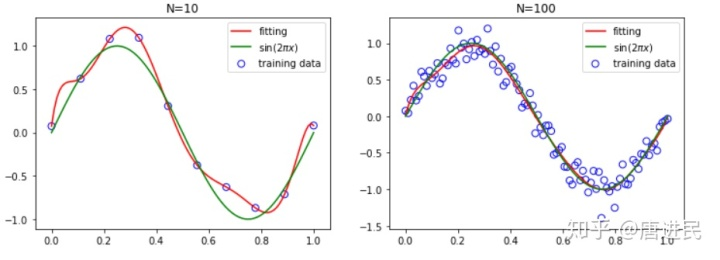代码如下：

fig = plt.figure(figsize=(12,4))
for i,N in enumerate([10,100]):
X_train,y_train = create_data(N)

plt.subplot(1,2,i+1)

poly = PolynomialFeatures(9)
X_train_ploy = poly.fit_transform(X_train)
X_test_ploy = poly.fit_transform(X_test)

lr = LinearRegression()
lr.fit(X_train_ploy,y_train)
y_pred = lr.predict(X_test_ploy)

plt.scatter(X_train,y_train,facecolor="none", edgecolor="b", s=50, label="training data")
plt.plot(X_test,y_pred,c="r",label="fitting")
plt.plot(X_test,y_test,c="g",label="$sin(2pi x)$")
plt.title("N={}".format(N))
plt.legend()
plt.show()

除了通过增加数据量的方式来减轻过拟合的影响，还可以通过正则化的方式来实现，这种技术就是在我们定义为误差函数增加一个惩罚项，是的多项式系数被有效的控制。均方误差函数修改后的形式为：其中，这种二次正则项的应用也叫作
山脊回归（Ridge Regression）,下面我们同样展示当 多项式的阶时，取时过拟合被压制的情况。如下图：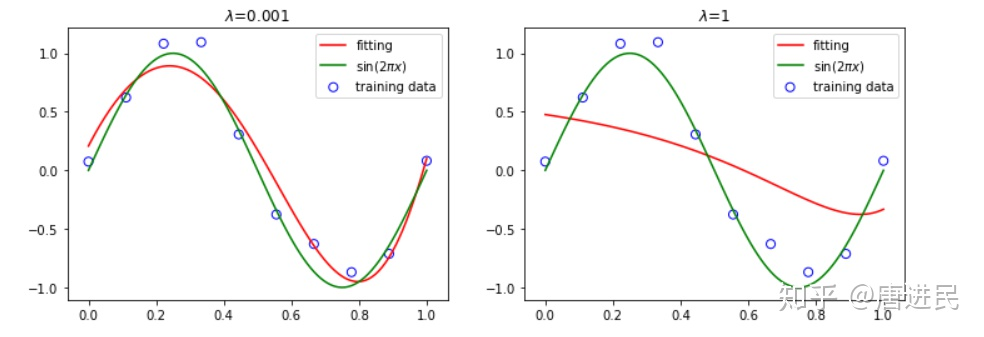代码如下：

fig = plt.figure(figsize=(12,4))
for i,lamb in enumerate([0.001,1]):
X_train,y_train = create_data(10)

plt.subplot(1,2,i+1)

poly = PolynomialFeatures(9)
X_train_ploy = poly.fit_transform(X_train)
X_test_ploy = poly.fit_transform(X_test)

lr = Ridge(alpha=(lamb/2))
lr.fit(X_train_ploy,y_train)
y_pred = lr.predict(X_test_ploy)

plt.scatter(X_train,y_train,facecolor="none", edgecolor="b", s=50, label="training data")
plt.plot(X_test,y_pred,c="r",label="fitting")
plt.plot(X_test,y_test,c="g",label="$sin(2pi x)$")
plt.title("$lambda$={}".format(lamb))
plt.legend()
plt.show()

可以看到，当过大的时候会过度抑制模型，所以根据模型复杂度来选择合适的对于模型最终的拟合结果有着重要的影响。

总结

在多项式拟合曲线的过程中，如果当我们模型复杂度被限制了，我们可以通过增加训练数据的方式防止模型的过拟合以及减轻被噪声数据的影响。同样当我们数据有限，想通过分析数据来选择模型的复杂度的话也可以通过正则化的方式来抑制模型的过拟合。当然也可以同时使用两种方式，合理的调整才能达到最佳拟合。

参考：PRML（Pattern-Recognition-and-Machine-Learning）

微信公众号：PyMachine展开全文• [Python] 多项式曲线拟合(Polynomial Curve Fitting)

万次阅读 多人点赞 2020-03-30 17:40:56
多项式曲线拟合Polynomial Curve Fitting实验目标实现过程- Step 1 ：生成观测集和目标函数- Step 2 ：比较不同阶数多项式的拟合效果- Step 3 ：通过增大数据规模改善过拟合现象- Step 4 ： 通过正则化改善过拟合...python 机器学习
• 本文介绍了MATLAB二维数据点RANSAC多项式拟合的方法与实现
• 文章目录一、 正交多项式曲线拟合1.曲线不经过起点与终点2.曲线经过起点与终点二、参考文献 一、 正交多项式曲线拟合 1.曲线不经过起点与终点 2.曲线经过起点与终点 二、参考文献 Trajectory Planning for Automatic...
• 2. 用高阶多项式函数拟合曲线； 3. 用解析解求解两种loss的最优解（无正则项和有正则项） 4. 优化方法求解最优解（梯度下降，共轭梯度）； 5. 用你得到的实验数据，解释过拟合。 6. 用不同数据量，不同超参数，...机器学习 python
• 多项式函数曲线拟合——最小二乘法

万次阅读 多人点赞 2019-05-13 12:49:01
多项式函数拟合的任务是假设给定数据由M次多项式函数生成，选择最有可能产生这些数据的M次多项式函数，即在M次多项式函数中选择一个对已知数据以及未知数据都有很好预测能力的函数。 最小二乘法（又称最小平方法）...多项式曲线拟合 统计分析
• 基于最小二乘法的自动分段多项式曲线拟合方法研究14 3 2014 1 科 学 技 术 与 工 程 Vol. 14 No. 3 Jan． 2014第 卷 第 期 年 月1671— 1815 (2014)03-0055-04 Science Technology and Engineering  2014 Sci. ...
• 非线性曲线拟合和多项式曲线拟合1.建模 2.非线性拟合求参数 3.完成拟合Fitting.m 1.建模 2.非线性拟合求参数 3.完成拟合 Fitting.m %% ================================================= %% 非线性曲线拟合和...非线性拟合
• % 多次多项式方程 % 误差项求和方程，这里是提供的值对应的函数值。 % 分别对不同系数求导数： ... % 最小二乘法求拟合曲线系数 for k=1:1:3 for g=1:1:4 sumdata1 = 0; for j=1:1:11 if g <= 3 ...
• //列主元LU分解 cout拟合函数的系数分别为:\n"; for(i=0;i cout["; } void f1(double *a,double *x) { int i,j,k; double temp; for(i=0;i for(j=0;j { temp=1; for(k=0;k temp*=x[j]; *(a+i*NUM+j)=temp; } } void...
• 本文使用 Zhihu On VSCode 创作并发布0 前言自动驾驶开发中经常涉及到多项式曲线拟合，本文详细描述了使用最小二乘法进行多项式曲线拟合的数学原理，通过样本集构造范德蒙德矩阵，将一元 N 次多项式非线性回归问题...
• 分别构造一次、二次、三次最小二乘多项式、指数函数和冥函数，画图并计算平方误差；
• 多项式曲线拟合（Polynomial Curve Fitting）

万次阅读 多人点赞 2016-06-05 10:18:16
多项式曲线拟合（Polynomial Curve Fitting）标签：监督学习多项式特征生成在机器学习算法中，基于针对数据的非线性函数的线性模型是非常常见的，这种方法即可以像线性模型一样高效的运算，同时使得模型可以适用于...多项式曲线拟合
• 函数关系式为： 注意：v(t)公式错误，系数c1后面没有t 已知起点(x0, v0, a0), 终点(x1, v1, a1), 两点之间，一段轨迹规划的起始时间是t0，结束时间是t1。 一般情况下，起点时刻默认为t0 = 0 ，根据五次多项式及其...
• 有数据如下，对其进行曲线拟合： x=[0;0.4;1.2;2;2.8;3.6;4.4;5.2;6;7.2;8;9.2;10.4;11.6;12.4;13.6;14.4;15]; y=[1;0.85;...使用二次多项式进行曲线拟合 close all; clc; clear; x=[0;0.4;1.2;2;2.8;matlab 曲线拟合 多项式曲线拟合...